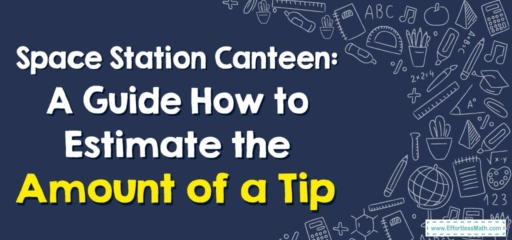# Space Station Canteen: A Guide How to Estimate the Amount of a Tip

Greetings, fellow space travelers!

For today's interstellar journey, we're not exploring galaxies or hunting for alien life forms, but instead, we're making a quick stop at the Space Station Canteen. And in space, like on Earth, it's important to understand the etiquette of tipping. So, let's take a look at how we can estimate the amount of a tip!## 1. Floating in Space: Understanding Tipping

Before we hop in the shuttle, let’s make sure we understand tipping:

• Tipping: This is a small amount of money that is given to a service worker, such as a waiter, in addition to the basic price.

## 2. The Space Mission: Estimating the Amount of a Tip

Now that we understand the concept of tipping, let’s head over to the canteen!

### Astro-Guide: Estimating the Amount of a Tip

#### Step 1: Determine the Total Bill

First, determine the total amount of your bill. This will be the amount before the tip is added.

#### Step 2: Choose the Percentage for the Tip

Decide the percentage you wish to tip. The common practice on Earth is $$15-20$$$$\%$$, but feel free to choose what feels right to you.

#### Step 3: Calculate the Tip

Finally, calculate the tip by multiplying the total bill by the tip percentage.

For instance, if your meal costs $$20$$ and you decide to tip $$15$$$$\%$$, how much is the tip?

1. Determine the Total Bill: The total bill is $$20$$.
2. Choose the Percentage for the Tip: You decide to tip $$15$$$$\%$$, which is $$0.15$$ as a decimal.
3. Calculate the Tip: Multiply the total bill by the tip percentage: $$20\times 0.15 = 3$$.

And there we have it, fellow space travelers! You’ve now mastered the art of estimating tips. Remember, whether we’re on Earth or in the far reaches of the cosmos, respect and courtesy are universal languages. Until our next interstellar adventure, happy space travels!

### What people say about "Space Station Canteen: A Guide How to Estimate the Amount of a Tip - Effortless Math: We Help Students Learn to LOVE Mathematics"?

No one replied yet.

X
30% OFF

Limited time only!

Save Over 30%

SAVE $5 It was$16.99 now it is \$11.99